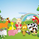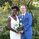# Grand Trend Forecasting - A Simple And Original Approachจำนวนเข้าชม 9571
Today we'll link time series forecasting with signal processing in order to provide an original and funny trend forecasting method, the post share lot of information, if you just want to see how to use the indicator then go to the section "Using The Indicator".

Time series forecasting is an area dealing with the prediction of future values of a series by using a specific model, the model is the main tool that is used for forecasting, and is often an expression based on a set of predictor terms and parameters, for example the linear regression (model) is a 1st order polynomial (expression) using 2 parameters and a predictor variable ax + b. Today we won't be using the linear regression nor the LSMA .

In time series analysis we can describe the time series with a model, in the case of the closing price a simple model could be as follows :

Price = Trend + Cycles + Noise

The variables of the model are the components, such model is additive since we add the component with each others, we should be familiar with each components of the model, the trend represent a simple long term variation of high amplitude, the cycles are periodic fluctuations centered around 0 of varying period and amplitude, the noise component represent shorter term irregular variations with mean 0.

As a trader we are mostly interested by the cycles and the trend, altho the cycles are relatively more technical to trade and can constitute parasitic fluctuations (think about retracements in a trend affecting your trend indicator, causing potential false signals).

If you are curious, in signal processing combining components has a specific name, "synthesis", here we are dealing with additive synthesis, other type of synthesis are more specific to audio processing and are relatively more complex, but could be used in technical analysis .

So what to do with our components ? If we want to trade the trend, we should estimate right ? Estimating the trend component involve removing the cycle and noise component from the price, if you have read stuff about filters you should know where i'am going, yep, we should use filters, in the case of keeping the trend we can use a simple moving average of relatively high period, and here we go.

However the lag problem, which is recurrent, come back again, we end up with information easier to interpret (here the trend, which is a simple fluctuation such as a line or other smooth curve) at the cost of decision timing, that is unfortunate but as i said the information, here the moving average output, is relatively simple, and could be easily forecasted right ? If you plot a moving average of high period it would be easier for you to forecast its future values. And thats what we aim to do today, provide an estimate of the trend that should be easy to forecast, and should fit to the price relatively well in order to produce forecast that could determine the position of future closing prices observations.

Estimating And Forecasting The Trend

The parameter of the indicator dealing with the estimation of the trend is length, with higher values of length attenuating the cycle and noise component in the price, note however that high values of length can return a really long term trend unlike a simple moving average , so a small value of length, 14 for example can still produce relatively correct estimate of trend.

here length = 14.

The rough estimate of the trend is t in the code, and is an IIR filter, that is, it is based on recursion. Now i'll pass on the filter design explanation but in short, weights are constants, with higher weights allocated to the previous length values of the filter, you can see on the code that the first part of t is similar to an exponential moving average with :

t(n) = 0.9t(n-length) + 0.1*Price

However while the EMA only use the precedent value for the recursion, here we use the precedent length value, this would just output a noisy and really slow output, therefore in order to create a better fit we add : 0.9*(t(n-length) - t(n-2length)), and this create the rough trend estimate that you can see in blue. On the parameters, 0.9 is used since it gives the best estimate in my opinion, higher values would create more periodic output and lower values would just create a rougher output.

The blue line still contain a residual of the cycle/noise component, this is why it is smoothed with a simple moving average of period length. If you are curious, a filter estimating the trend but still containing noisy fluctuations is called "Notch" filter, such filter would depending on the cutoff remove/attenuate mid term cyclic fluctuations while preserving the trend and the noise, its the opposite of a bandpass filter.

In order to forecast values, we simply sum our trend estimate with the trend estimate change with period equal to the forecasting horizon period, this is a really really simple forecasting method, but it can produce decent results, it can also allows the forecast to start from the last point of the trend estimate.

Using The Indicator

We explained the length parameter in the precedent section, src is the input series which the trend is estimated, forecast determine the forecasting horizon, recommend values for forecast should be equal to length, length/2 or length*2, altho i strongly recommend length.

here length and forecast are both equal to 14 .

The corrective parameter affect the trend estimate, it reduce the overshoot and can led to a curve that might fit better to the price.

The indicator with the non corrective version above, and the corrective one below.

The source parameter determine the source of the forecast, when "Noisy" is selected the source is the blue line, and produce a noisy forecast, when "Smooth" is selected the source is the moving average of t, this create a smoother forecast.

The width interval control...the width of the intervals, they can be seen above and under the forecast plot, they are constructed by adding/subtracting the forecast with the forecast moving average absolute error with respect to the price. Prediction intervals are often associated with a probability (determining the probability of future values being between the interval) here we can't determine such probability with accuracy, this require (i think) an analysis of the forecasting distribution as well as assumptions on the distribution of the forecasting error.

Finally it is possible to see historical forecasts, that is, forecasts previously generated by checking the "Show Historical Forecasts" option.

Examples

Good forecasts mostly occur when the price is close to the trend estimate, this include the following highlighted periods on AMD 15TF with default settings :

We can see the same thing at the end of EURUSD :

However we can't always obtain suitable fits, here it is isn't sufficient on BTCUSD :

We can see wide intervals, we could change length or use the corrective option to get better results, another option is to use a log scale.

We will end the examples with the log SPX , who posses a linear trend, so for example a linear model such as a linear regression would be really adapted, lets see how the indicator perform :

Not a great fit, we could try to use an higher length value and use "Smooth" :

Most recent fits are quite decent.

Conclusions

A forecasting indicator has been presented in this post. The indicator use an original approach toward estimating the trend component in the closing price. Of course i should have given statistics related to the forecasting error, however such analysis is worth doing with better methods and in more advanced environment allowing for optimization.

But we have learned some stuff related to signal processing as well as time series analysis, seeing a time series as the sum of various components is really helpful when it comes to make sense of chaotic and noisy series and is a basic topic in time series analysis.

You can see that in this new year i work harder on the visual of my indicators without trying to fall in the label addict trap, something that i wasn't really doing before, let me know what do you think of it.

สคริปต์โอเพนซอร์ซ

จากใจ TradingView ผู้เขียนสคริปต์นี้ได้เผยแพร่เป็นโอเพนซอร์ส เพื่อให้ผู้ค้าสามารถเข้าใจและตรวจสอบได้ ยินดีด้วย! คุณสามารถใช้มันได้ฟรี แต่การใช้โค๊ดนี้ซ้ำเพื่อการเผยเเพร่ อยู่ภายใต้ กฎของเรา คุณสามารถตั้งเป็นรายการโปรดเพื่อใช้กับชาร์ตได้

ต้องการที่จะใช้สคริปต์นี้บนชาร์ตใช่ไหม?
Patreon: https://www.patreon.com/alexgrover

You can also check out some of the indicators I made for luxalgo : https://www.tradingview.com/u/LuxAlgo/#published-scripts

## ความคิดเห็นThis is incredible! I can see Alex you put a lot of efforts in it which I really appreciated. Thanks again for your detailed precise explanation with clear charts indication.

Thank you so much Alex =D
ตอบกลับICEKI
@ICEKI, Thx for acknowledging the efforts :) Much appreciated
ตอบกลับalexgrover
@alexgrover, You're welcome you definitely deserve it =D
ตอบกลับdo you have recommended buy and sell signals for this indicator?
ตอบกลับI'm afraid I will have to read this through another 5 times to be sure to get it all!! ;-)
As always, great work, Alex!!
Gr, JD.
ตอบกลับDuyck
@Duyck, Thx for the support, if you need to right it back several times then it probably means that i failed my explanation, will try to be more concise and clear in the future, thanks for your feedback.
ตอบกลับalexgrover
@alexgrover, I'm afraid, chances are, it has more to do with my rusty match skills than with your excellent explanation skills!! ;-)
ตอบกลับ